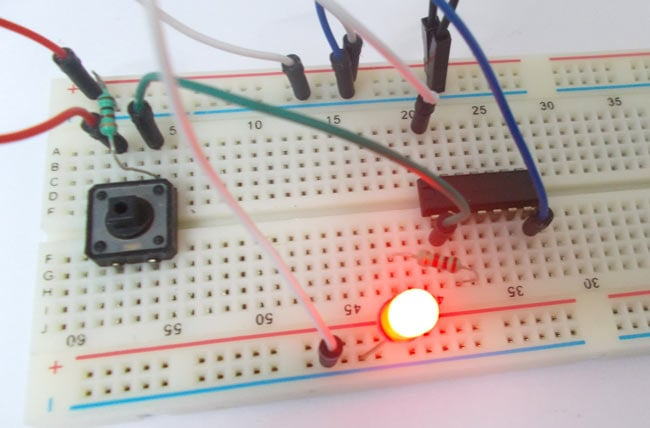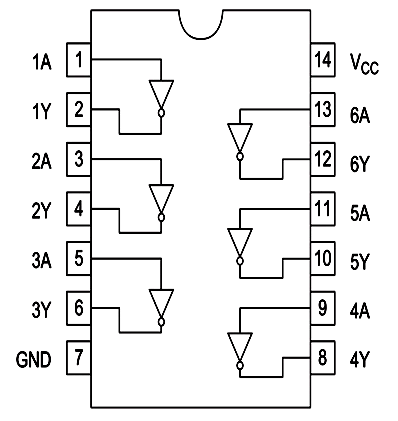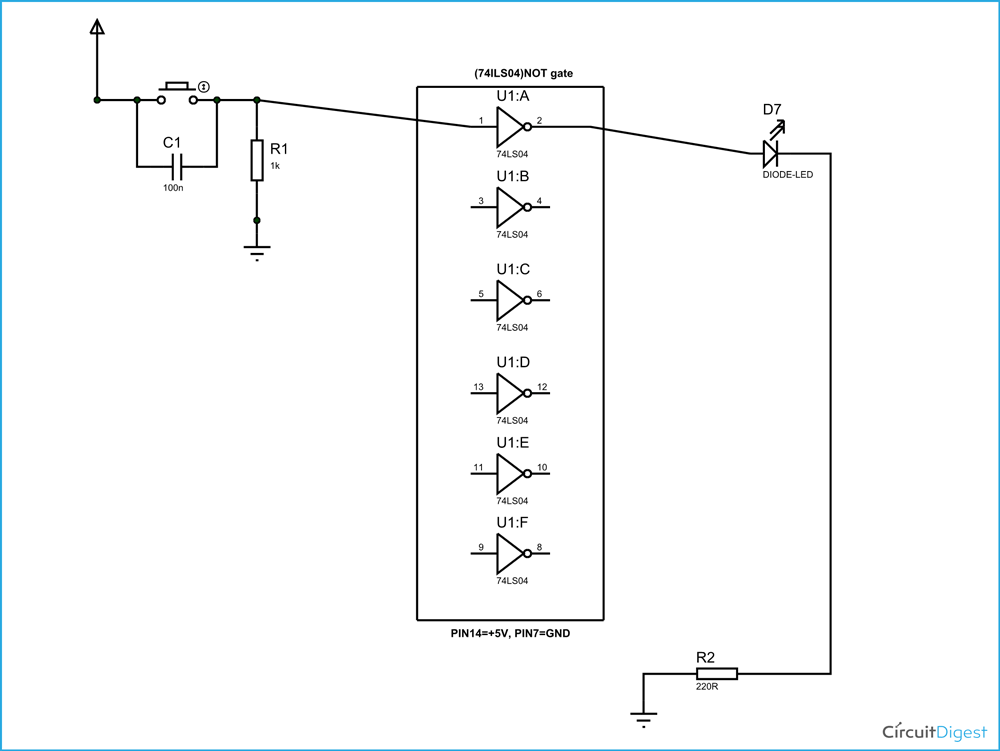# NOT Gate

Published  August 24, 2015   0NOT gate is a digital logic gate, designed for arithmetic and logical operations, every electronic student must have studied this gate is his/her career. This gate is mainly used in applications where there is a need for mathematical calculations. So in calculators, computers and many digital applications use this gate.

NOT gates are simply inverters. They simply invert the input logic for the output. Here we are going to use 74LS04 IC for demonstration. This IC has 6 NOT gates in it. These SIX gate are connected internally as shown in below figure.These gates have limitations for working voltage and input logic frequency. When these limitations are not considered the IC may be damaged permanently, so one should pay attention while selecting the logic gates.

### Components Required

Power supply (5v)

1K, 220Ω resistor

74LS04 HEX NOT GATE IC

1 LED

Button

100nF capacitor

Connecting wires

### Circuit Diagram and Working Explanation

The truth table of NOT gate is show below

Y = A (bar)

 Input Output A Y L H H L

H – High Logic Level

L – Low Logic Level

As of circuit diagram an inverter gate has one output for one input. As by the truth table, the output of NOT gate will be high when the input is low. The output of NOTgate should be low when the input is high. So the NOT gate provides output which is inverted logic of input.

In this NOT gate circuit we are going to pull down both input of a gate to ground through a 1KΩ resistor.  And then the input is connected to power through a button.So when the button is pressed the corresponding pin of gate goes high. So with this button we can realize the truth table of NOT gate. When the button is pressed the input will go high, with this the output will go low and so the LED should be off. When the button is released the input will go LOW, with this the OUTPUT will go HIGH and so the LED should be ON.

These pull down resistor are necessary as the chosen CHIP is a positive edge triggering one. If the resistor is ignored the circuit might generate unpredictable results.

The capacitor here is for neutralizing the bouncing effect of button. Although the capacitor here is not compulsory, putting them might smooth the working of NOT gate.

Video